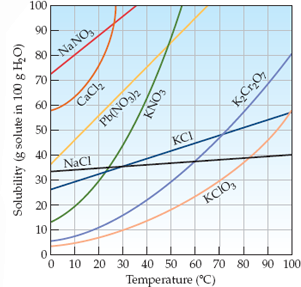×
Get Full Access to Introductory Chemistry - 5 Edition - Chapter 13 - Problem 36p
Get Full Access to Introductory Chemistry - 5 Edition - Chapter 13 - Problem 36p

×

# Solved: Refer to Figure 1 determine whether each of the given amounts of solid willISBN: 9780321910295 34

## Solution for problem 36P Chapter 13

Introductory Chemistry | 5th Edition

• Textbook Solutions
• 2901 Step-by-step solutions solved by professors and subject experts
• Get 24/7 help from StudySoup virtual teaching assistantsIntroductory Chemistry | 5th Edition

4 5 1 239 Reviews
25
4
Problem 36P

Refer to Figure 1 determine whether each of the given amounts of solid will completely dissolve in the given amount of water at the indicated temperature.

(a) 45.0 g CaCl2 in 105 g of water at 5 °C

(b) 15.0 g KClO3 in 115 g of water at 25 °C

(c) 50.0 g Pb(NO3)2 in 95.0 g of water at 10 °C

Figure 1 Solubility of some ionic solids as a function of temperatureStep-by-Step Solution:
Step 1 of 3

Problem 36P

Refer to Figure 1 determine whether each of the given amounts of solid will completely dissolve in the given amount of water at the indicated temperature.

(a) 45.0 g CaCl2 in 105 g of water at 5 °C

(b) 15.0 g KClO3 in 115 g of water at 25 °C

(c) 50.0 g Pb(NO3)2 in 95.0 g of water at 10 °C

Figure 1 Solubility of some ionic solids as a function of temperatureSolution: Here, we are going to identify if the given amounts of solid will dissolve completely in the given amount of water at the indicated temperature.

Step1:

Solubility of a substance is its maximum amount that can be dissolved in a specified amount of solvent. It depends upon the nature of solute and solvent as well as temperature and pressure.

Step2:

1. From figure 1, it is clear that the solubility of CaCl2 at 5 oC is about 60 g per 100 g of water.

Thus, 45.0 g of  CaCl2 will dissolve completely in 105 g water at 5 oC.

b)        From figure 1, it is clear that the solubility of KClO3 at 25 oC is about 8 g per 100 g of

water.

So, for dissolving 15.0 g of KClO3, amount of water required = (100/8) x 15 = 187 g

water

Thus, 15.0 g of  KClO3 will not dissolve in 115 g water at 25 oC.

c)        From figure 1, it is clear that the solubility of Pb(NO3)2 at 10 oC is about 45 g per 100 g

of water.

Thus, 50.0 g of  Pb(NO3)2 will not dissolve in 95.0 g water at 10 oC.

------------------------------

Step 2 of 3

Step 3 of 3

##### ISBN: 9780321910295

Since the solution to 36P from 13 chapter was answered, more than 1704 students have viewed the full step-by-step answer. The answer to “Refer to Figure 1 determine whether each of the given amounts of solid will completely dissolve in the given amount of water at the indicated temperature.(a) 45.0 g CaCl2 in 105 g of water at 5 °C(b) 15.0 g KClO3 in 115 g of water at 25 °C(c) 50.0 g Pb(NO3)2 in 95.0 g of water at 10 °CFigure 1 Solubility of some ionic solids as a function of temperature” is broken down into a number of easy to follow steps, and 70 words. The full step-by-step solution to problem: 36P from chapter: 13 was answered by , our top Chemistry solution expert on 05/06/17, 06:45PM. This textbook survival guide was created for the textbook: Introductory Chemistry, edition: 5. This full solution covers the following key subjects: Water, temperature, figure, given, ionic. This expansive textbook survival guide covers 19 chapters, and 2046 solutions. Introductory Chemistry was written by and is associated to the ISBN: 9780321910295.

Unlock Textbook Solution